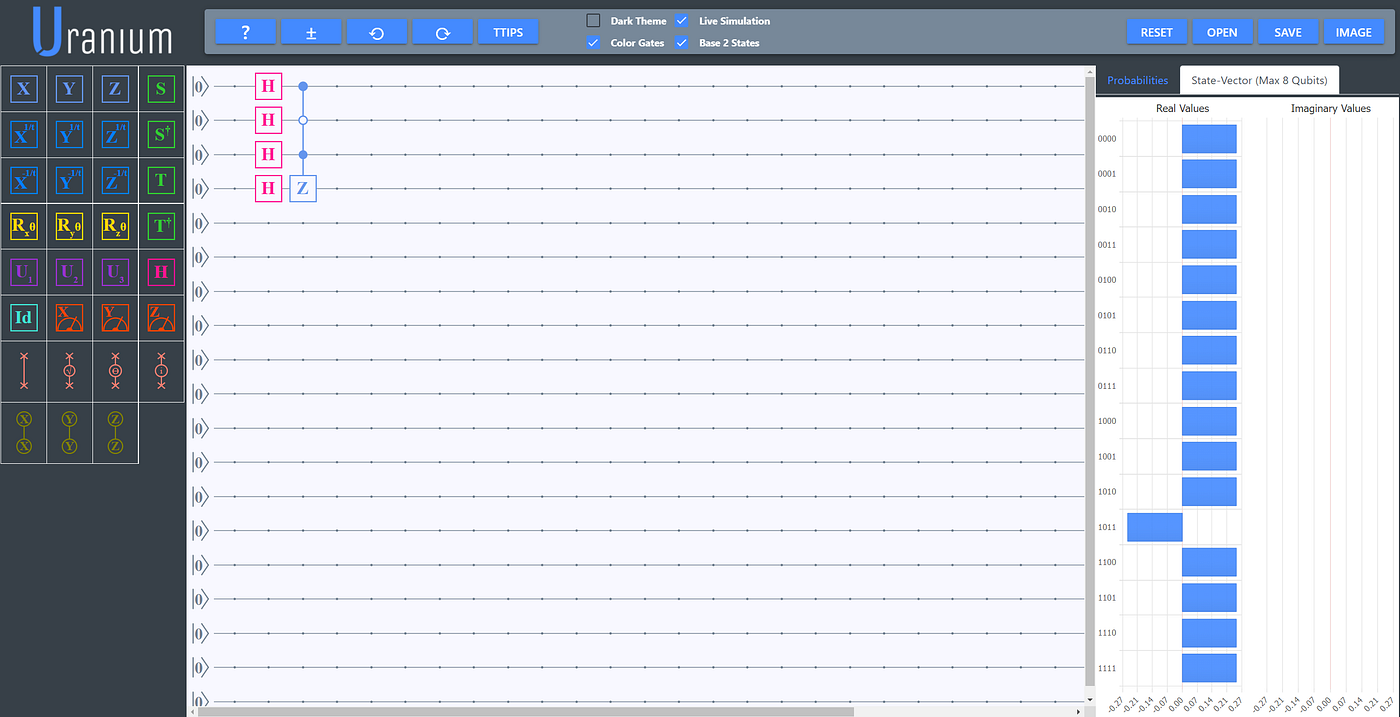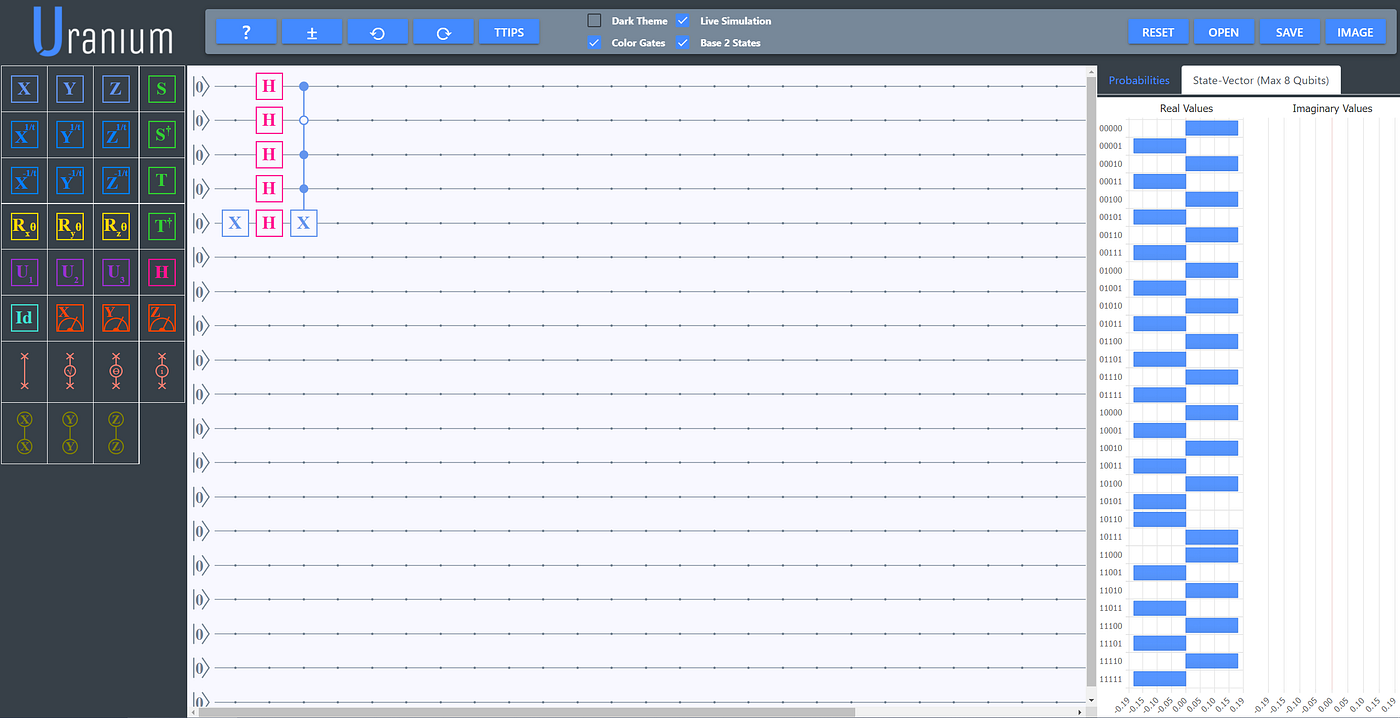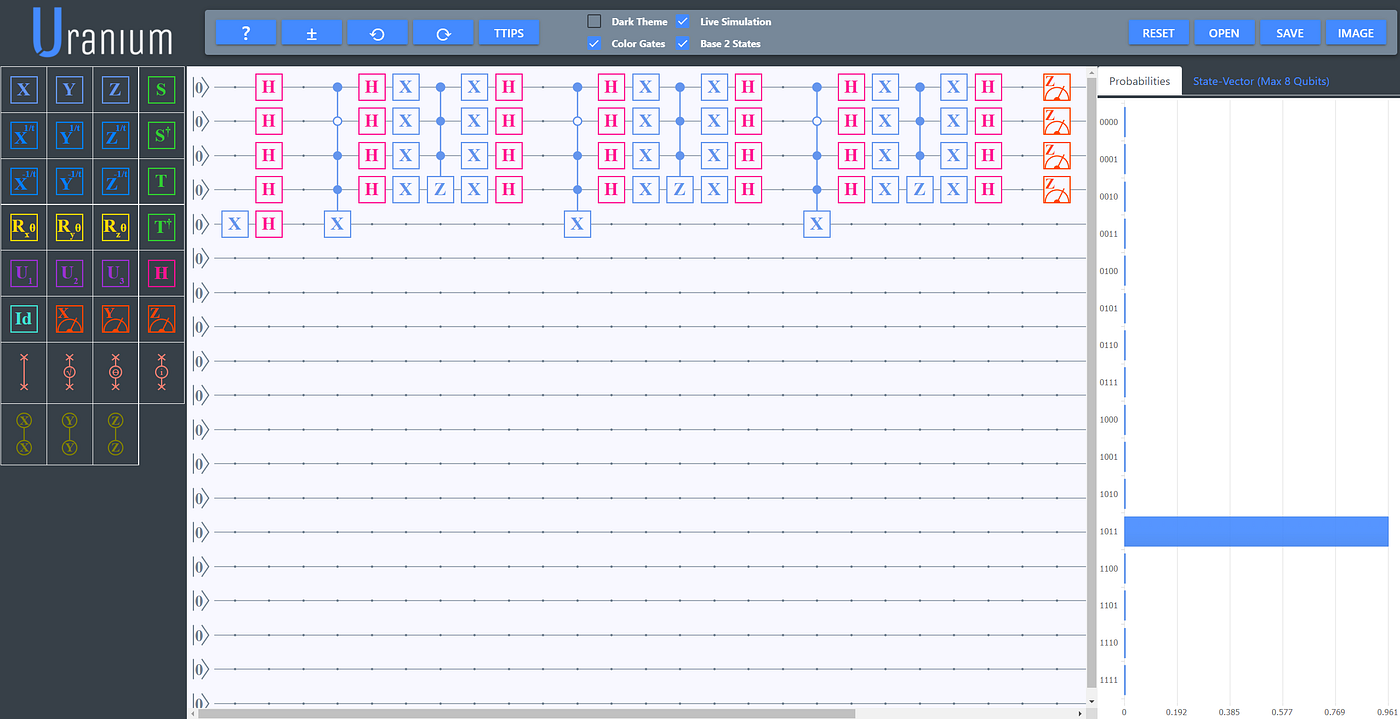# Simple Implementation of Grover Search on Uranium

Ok, so if you’re reading this article I’m assuming you’ve heard of Grover’s search algorithm (if not I’ll explain), but what the hell is Uranium?

Uranium is this cool quantum computing platform that features a circuit editor, visualization tools and a built in simulator that runs in browser. I wrote about it here.

Grover Search is a quantum search algorithm. It’s famous and I’ll explain why. In the classical world, if you have an unordered list (or DB) of length N and you want to find an element that satisfies a condition, you must look at every element at least once, to check the condition. This means you must look at N elements so classical search algorithms are order N. But using Grover’s algorithm you can find the element by checking the condition sqrt(N) times.

This quantum algorithm is quadratically faster than any classical search algorithm. But, as always, there’s a catch. It doesn’t search a list or a DB, but rather it searches a superposition. To get an item from the element in the superposition we’d need something called a QRAM, but I digress…

It goes like this:

1. Start from a uniform distribution across all the space you want to search — all elements of the superposition have the same probability.
2. Mark the elements you want to find by changing their sign (don’t worry if you don’t get it, we’ll see an example)
3. Apply an operator that will flip all probabilities across their mean. This is called the diffusion operator and its the same for any implementation/condition.
4. Calculate how many times r = [pi*sqrt(N)/4]. Repeat steps (2) and (3) r times.

# Our implementation

In this example we’ll search all numbers of 4 bits, 0 to 15. In binary this is |0000> to |1111>. This means the length of our search space is N=16. We’ll try to find the number 11 or |1011>. This means that our search condition will be |x> == |1011>.

## Step one: uniform distribution

This is the easy part. Our space uses just four qubits. We apply a Hadamard gate to every qubit to bring us to the full superposition in which every element has the same probability.

## Step two: mark the states to be found

What do I mean by “change the sign”? Ignoring the normalization factor the superposition we have from step 1 is

We are searching for 11 so we want to change the sign for it and have

We can do that in two ways. We could just apply a controlled Z to do that. You can switch to the “State vector” tab in the results pane to see what happens to the superposition.Mark the state |1011> using a controlled Z gate.

But there is a big question here: If we know what we are searching for and we know how to change it’s sign then why are we searching for it? That’s why using a controlled Z would not be used in a real implementation. It turns out that there’s another way to change the sign: by using phase kick-back.

Phase kick-back is a phenomenon that happens in quantum computing. You can start by having an ancilla qubit in the |->state. You want to entangle it only whit the sates you are searching for. Note that you don’t have to know what is the state you are searching. You just have to have an oracle — which encodes the search criteria — that takes the sates you are looking for and entangles them to the ancilla qubit. The effect of this entanglement is to flip the sign of the desired state. This is called phase kick-back.

In our case the oracle is quite simple: it entangles the state |1011> with the ancilla. In real scenarios, like searching for a solution to the travelling salesman problem the oracle would be more complex.Mark the state |1011> using phase kick-back

Notice in the above image we’ve added a fifth qubit and set it to |-> by adding an X and H gate. Then we added the controlled-X gate that entangles only |1011> to this qubit. Also notice in the results pane that the sate-vector has become more complex because of this new qubit. But if you look carefully you can see that the sates starting in |1011_> are flipped in comparison with the others. Ignoring the normalization factor the full state is

## Step three: inversion about the mean

To invert about the mean we just use the diffusion operator. This is a standard construction that only depends on the number of qubits.

To get the result we’ll only measure the first four qubits.

You can see that the probability of measuring |1011> is almost 50%. But we can do better.

## Step four: rinse and repeat

We now compute the number of repetitions needed. r=[pi*sqrt(16)/4]=3. We need to apply the oracle and diffusion operator three times in total.Applying the oracle and diffusion operator three times

Now the probability of measuring |1011> is more than 96%. Adding the operators again will decrease this probability. You can try it yourself.

# Conclusion

There you go. We’ve found a number (with high probability) in a superposition of 16 possibilities, by applying the search condition 3 times.

Admittedly it was a trivial case. We already knew the exact number we were searching for. But this works for searching for solutions to complex problems. We just need to encode the problem in qubits/superpositions and come up with a good oracle to reflect the search criteria. The rest stays the same.

I have a plan to write about a more complex search. But meanwhile you can try building the algorithm yourself. Just go to https://uranium.transilvania-quantum.org/ and give it a go.

--

--